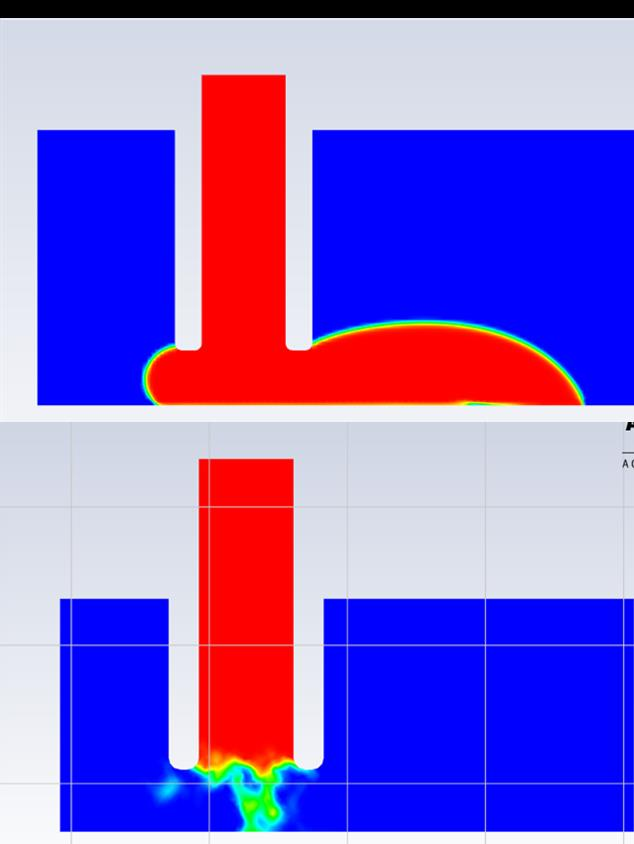# VOF model for slurry deposition trough an extruder

Dear,
I, as a completely new guy to Ansys, started building up a model in fluent to simulate the slurry (fluid) deposition through an extruder. First I started to get to know the software via the tutorials in VOF modelling and some overall tutorials. Then I started with a 2D-model to try it out in a simplified model. After this gave me some decent results, I started rebuilding this model in 3d with the same parameters. But when I run this, the calculated VOF surface doesn't look realistic at all with a slurry being so vicious. Especially compared to the 2D-model. And next to that it gives the floating-point error after around 40 iterations. In the attachments of this topic, I uploaded one file containing the two summary files of the model. First, the 2D model summary is given, behind that the 3D model summary

Would be pleased to have someone that could look at it and give me some tips on what to do next to get some valid results.

Sincerely,

Guus

• edited April 20
as an Ansys employee I do not download attachments. Slow settling or no settling slurries can be modeled as non Newtonian fluid. If you require sir as surrounding then simple two phase flow with the slurry with adjusted properties and non Newtonian viscosity
• edited April 20
• Dear Amine,

The 3D model now consists of a nozzle at a fixed distance above a substrate. The substrate is moving in one direction to model the slurry deposition during printing. To test the model, the slurry now just has a fixed viscosity of 1 pas/s, this will later be changed to a non Newtonian viscosity.

As a time step I use a function that changes the time step per iteration: IF(iter<=600, 1 [s] *10**-(10-iter*0.01), 0.0001 [s]) with a total of 1700 iterations.

Down here I put the text I wrote the settings of the 3d model (the same as in the attachments).

Fluent
Version: 3d, dp, pbns, vof, lam, transient (3d, double precision, pressure-based, VOF, laminar, transient)
Release: 20.1.0
Title:

Models
------

Model                        Settings
---------------------------------------------------------
Space                        3D
Viscous                      Laminar
Heat Transfer                Disabled
Solidification and Melting   Disabled
Species                      Disabled
Coupled Dispersed Phase      Disabled
NOx Pollutants               Disabled
SOx Pollutants               Disabled
Soot                         Disabled
Mercury Pollutants           Disabled
Structure                    Disabled

Material Properties
-------------------

Material: slurry (fluid)

Property                        Units     Method     Value(s)
-------------------------------------------------------------
Density                         kg/m3     constant   7260
Cp (Specific Heat)              j/kg-k    constant   1006.43
Thermal Conductivity            w/m-k     constant   0.0242
Viscosity                       kg/m-s    constant   1
Molecular Weight                kg/kmol   constant   28.966
Thermal Expansion Coefficient   1/k       constant   0
Speed of Sound                  m/s       none       #f

Material: air (fluid)

Property                        Units     Method     Value(s)
---------------------------------------------------------------
Density                         kg/m3     constant   1.225
Cp (Specific Heat)              j/kg-k    constant   1006.43
Thermal Conductivity            w/m-k     constant   0.0242
Viscosity                       kg/m-s    constant   1.7894e-05
Molecular Weight                kg/kmol   constant   28.966
Thermal Expansion Coefficient   1/k       constant   0
Speed of Sound                  m/s       none       #f

Material: aluminium (solid)

Property               Units    Method     Value(s)
---------------------------------------------------
Density                kg/m3    constant   2719
Cp (Specific Heat)     j/kg-k   constant   871
Thermal Conductivity   w/m-k    constant   202.4

Cell Zone Conditions
--------------------

Zones

name    id   type
------------------
fluid   3    fluid

Setup Conditions

fluid

Condition       Value
---------------------
Frame Motion?   no
Mesh Motion?    no

Boundary Conditions
-------------------

Zones

name              id   type
--------------------------------------
wall-fluid        1    wall
symmetry-plane    6    symmetry
pressure-outlet   7    pressure-outlet
moving-wall       8    wall
velocity-inlet    9    velocity-inlet

Setup Conditions

wall-fluid

Condition                  Value
-----------------------------------------------------------------
Wall Motion                0
Shear Boundary Condition   0
Contact Angles             (((constant . 2.0943948) (profile  )))

symmetry-plane

Condition   Value
-----------------

pressure-outlet

Condition   Value
-----------------

moving-wall

Condition                                            Value
-------------------------------------------------------------------------------------------
Wall Motion                                          1
Shear Boundary Condition                             0
Define wall motion relative to adjacent cell zone?   yes
Velocity Magnitude (m/s)                             0.01
Contact Angles                                       (((constant . 1.5707963) (profile  )))

velocity-inlet

Condition                  Value
--------------------------------
Velocity Magnitude (m/s)   0.01

Solver Settings
---------------

Equations

Equation          Solved
------------------------
Flow              yes
Volume Fraction   yes

Numerics

Numeric                         Enabled
---------------------------------------
Absolute Velocity Formulation   yes

---------------------------------------------
Time Step (s)                   2.5118864e-09
Max. Iterations Per Time Step   20

Non-Iterative Solver Factors

Variable          Max. Corrections   Correction Tolerance   Residual Tolerance   Relaxation Factor
--------------------------------------------------------------------------------------------------
Pressure          10                 0.25                   0.0001               1
Momentum          5                  0.05                   0.0001               1
Volume Fraction   5                  0.05                   0.0001               1

Linear Solver

Variable          Solver Type   Termination Criterion   Residual Reduction Tolerance
------------------------------------------------------------------------------------
Pressure          F-Cycle       0.1
X-Momentum        Flexible      0.1                     0.7
Y-Momentum        Flexible      0.1                     0.7
Z-Momentum        Flexible      0.1                     0.7
Volume Fraction   Flexible      0.1                     0.7

Pressure-Velocity Coupling

Parameter   Value
---------------------------
Type        Fractional Step

Discretization Scheme

Variable          Scheme
-----------------------------
Pressure          PRESTO!
Momentum          QUICK
Volume Fraction   Compressive

Solution Limits

Quantity                    Limit
---------------------------------
Minimum Absolute Pressure   1
Maximum Absolute Pressure   5e+10
Minimum Temperature         1
Maximum Temperature         5000

I hope you now have a general idea of the problem and that you can help me further.

Sincerely,

Guus van Wezel

• edited April 21

What is now the problem?

• Dear Amine,

The problem is that the results of the 2D and 3D models are very different. Where the 2D model gives a realistic flow of the slurry, the 3D model does not give a realistic flow at all. This is shown in the figure below.Since the input parameters for both models are the same, I can't locate the problem within the model.

Sincerely,

Guus